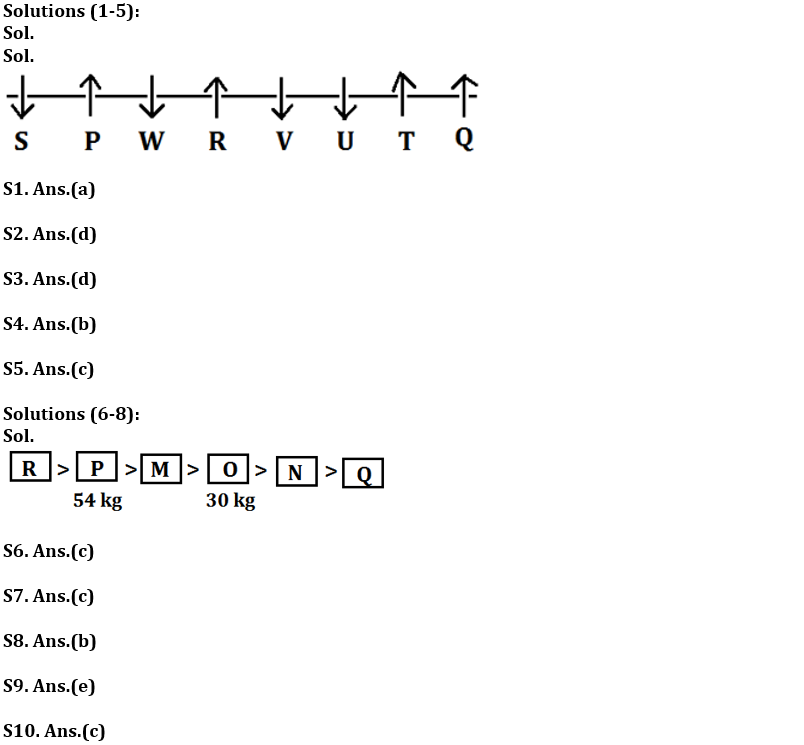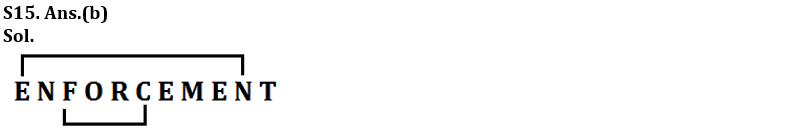Latest Banking jobs   »   Reasoning Quiz For RBI Grade B...

# Reasoning Quiz For RBI Grade B Phase 1 2023 – 07th May

Directions (1-5): Study the following information carefully and answer the questions given below:
Eight persons are sitting in a row, in such a way some of them are facing south while some are facing north. V sits third to the left of Q. U is not an immediate neighbour of Q. P sits third to the right of V. W sits to the immediate right of P. W does not sits at any of the extreme end of the line. Only one person sits between R and U. P sits second to the left of R. S sits second to the right of W. Both the immediate neighbour of P faces the same direction to each other. Both the immediate neighbour of V faces the opposite direction to each other. S faces the same direction as W. T faces the same direction as R. V faces same direction as U. T faces north.

Q1. Who among the following sits immediate right of V?
(a) R
(b) T
(c) U
(d) S
(e) None of these

Q2. How many persons sit left of W?
(a) Two
(b) Four
(c) Three
(d) More than four
(e) None

Q3. Who among the following is an immediate neighbour of S?
(a) R
(b) W
(c) Q
(d) P
(e) None of these

Q4. Which of the following statement is true about Q?
(a) S is an immediate neighbor of Q
(b) W faces opposite direction to Q
(c) Only two persons are sitting between Q and R
(d) U sits third to the left of Q
(e) None of these

Q5. What is the position of T with respect to R?
(a)Second to the right
(b) Third to the left
(c) Third to the right
(d) Immediate left
(e) None of these

Directions (6-8): Read the given information carefully and answer the given questions.
Six boxes M, N, O, P, Q, and R are placed horizontally in a room, each box is of different weight. Weight of box R is a perfect cube of a number. Box M is heavier than O but lighter than R. Only one box is lighter than N. P is heavier than Q and its weight is 54 kg. O is not the lightest box. Weight of third lightest box is 30 kg. Not more than three boxes are lighter than M.

Q6. Which of the following box is lightest?
(a) Box O
(b) Box M
(c) Box Q
(d) Box R
(e) None of These

Q7. What can be the possible weight of box R?
(a) 27 Kg
(b) 8 Kg
(c) 64 Kg
(d) 55 kg
(e) None of these

Q8. Which of the following is the second heaviest box?
(a) Box O
(b) Box P
(c) Box M
(d) Box Q
(e) Box R

Q9. If in the number 683297514, all digits are arranged in increasing order from right to left than how many digits remain the same place in the new arrangement?
(a) Three
(b) Four
(c) none
(d) Two
(e) One

Q10. Which of the following elements should come in a place of ‘?’

MN4 OL6 QJ8 ?

(a) TG12
(b) SP12
(c) SH10
(d) LO12
(e) None of these

Q11. Which of the following letter is 2nd to the right of the letter which is 7th from the right end, if each of the alphabets in the word ‘INVESTIGATION’ is arranged in alphabetical order from left to right?
(a) O
(b) S
(c) I
(d) G
(e) N

Q12. What will come in place of question-mark (?) in the following series?
AZ, BY, CX, ?
(a) WD
(b) DW
(c) DE
(d) DX
(e) DU

Q13. There are five persons A, B, C, D and E sit in a row facing north, B sits second to the left of A, E sits second to the right of D. Who among the following sits exactly middle in the row?
(a) E
(b) B
(c) D
(d) C
(e) Can’t be determine

Q14.In a row of children facing North, B is 12th from the right end and is fifth to the right of A who is 15th from the left end. Then, how many children are there in the row?
(a) 31
(b) 40
(c) 26
(d) 42
(e) None of these

Q15. How many pairs of letters are there in the word ‘ENFORCEMENT’, each of which have as many letters between then in the word as they have between then in the English alphabet?
(a)One
(b)Two
(c)Three
(d)None
(e) More than Three

SolutionsS11. Ans.(a)
Sol. original word- INVESTIGATION
After rearrangement- AEGIIINNOSTTV

S12. Ans.(b)

S13. Ans.(e)

S14. Ans.(a)
Sol. Position of B from right is = 12
Position of A from left is = 15
According to the question B is fifth to the right of A, that means position of B from left is 20
So, No. of children in the row is= 12+20-1= 31## FAQs

### What is the selection process of the Bank Clerk?

There are 4 sections in the RBI Grade B Phase 1 Exam i.e. English Language, General Awareness, Quantitative Aptitude & Reasoning.

#### Congratulations!Union Budget 2023-24: Free PDF